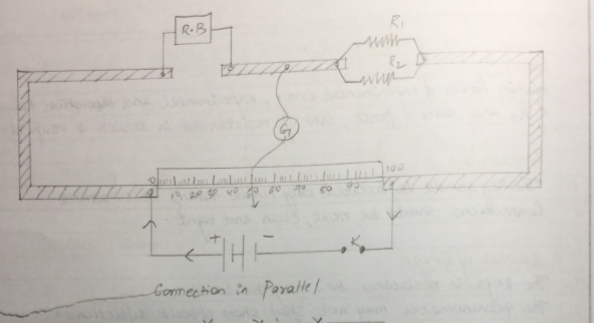# Verifying laws of combination (parallel) of resistance using meter bridge

Aim: To verify laws of combination (parallel) of resistance using meter bridge .

Apparatus: a meter bridge , a leclanche cell , a galvanometer , a jockey , a jockey , a resistance box , two resistance wire , connecting wires , set square .

Theory

The resistance (r) of a resistance wire or coil is given by r=(100-l)/L *R where R is the resistance from resistance box in the left gap and L is the length of meter bridge wire from O end upto balance point.

When r1 and r2 are connected in parallel , then their combined resistance

Rp = r1r2 /r1+r2

Procedure

1. Mark the two resistance wires as r1 and r2
2. Connect the two coils r1 and r2 in parallel as shown in fig. in the right gap of meter bridge and find the resistance of this combination . Take at least three set of observation

Result

Within limit of experimental error , experimental and theoretical values of Rp are same .

Hence law of resistance in parallel are verified .

Precaution

The key should be inserted only while taking observation .

Connection should be neat and tight .

Source of error

The galvanometer may not show opposite deflection

The key in the resistance box may be close .

OBSERVATION TABLE

Value of r1

 Resistance of R.B. (ohm) Balance L (cm) (100-l) (cm) [(100-l)*R]/L Mean r1 0.5 59 41 0.347 0.6393 1 37 63 0.587 2 33 67 0.985

Value of r2

 Resistance of R.B. (ohm) Balance L (cm) (100-l) (cm) [(100-l)*R]/L Mean r2 0.5 83.2 16.8 0.1009 1.729 1 80.8 19.2 4.208 2 69.4 30.6 0.881

Value of rp

 Resistance of R.B. (ohm) Balance L (cm) (100-l) (cm) [(100-l)*R]/L Mean rp 0.1 27 73 0.27 0.36 0.2 32 68 0.42 0.5 56 44 0.39

Calculations

Rp = (R1R2)/R1+R2 = (0.693×1.729)/(0.693+1.729) = 0.4667 ohm

Experimental Error = [(0.36-0.4667)/(0.36)]*100 = 29.6%Viva question

1. How does resistance change in parallel combination ?

Resistance decrease in parallel combination

2. explain the decrease of resistance in parallel combination ?

Effective area of cross section increases as r x 1/A , resistance decreases .

3. Why should jockey not be pressed too hard on wire while sliding ?

Sliding the jockey with a hard press will scratch the wire and make its thickness non-uniform .Resistance per unit length of wire will be inconsistent as resistance depends on area of cross section .

4. What is the resistance of an open key ? Explain.

Open key has infinite resistance as it makes current zero (R=V/I)

5. Define unit of resistance .

SI unit of resistance is OHM or volt per ampere . 1 Ohm is the resistance of a conduction carrying 1 ampere current when potential difference maintained across its end is 1 volt .

You can also get Class XII Practicals on BiologyPhysics, and Physical Education.

## 8 Replies to “Verifying laws of combination (parallel) of resistance using meter bridge”

1.Shiny Saha says:

Thanks a lot …😊😊😊

2.Zaid imtiaz says:

***** (5 star)
Very good

3.Anne says:

Super….it’s really useful

4.Shreshth tyagi says:

It’s very great site to do practicle

5.Tripti says:

Wow that’s great

6.Neelam says:

Thanks for the observation table

7.Sinchana shetty says:

If resistance in the right gap of meter bridge increased, what happens to the balancing length? And why?

8.Nitesh Kumar says:

Tq so much for this